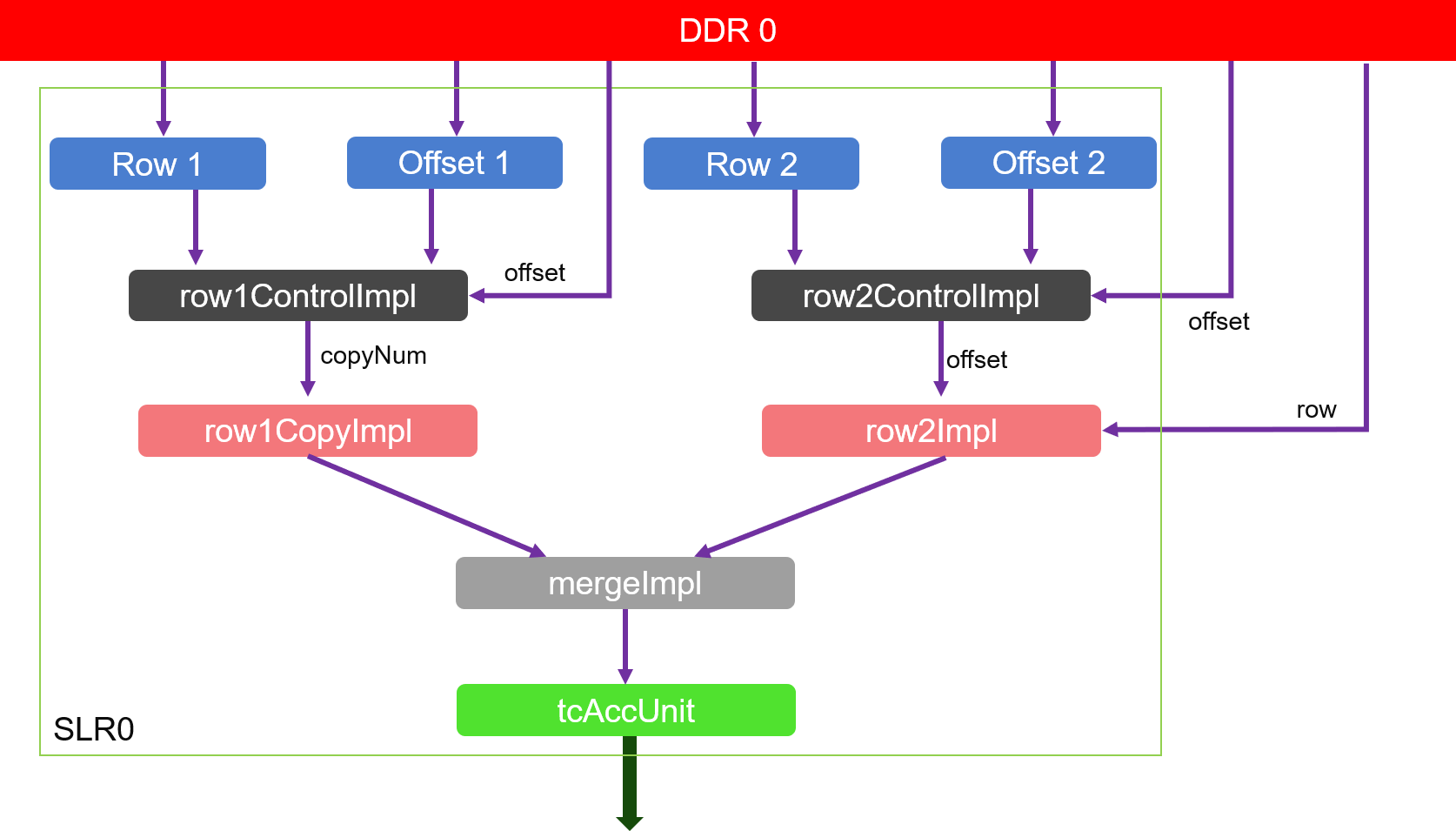# Internal Design of Triangle Counting¶

## Overview¶

Triangle counting is a community detection graph algorithm that is used to determine the number of triangles passing through each node in the graph. A triangle is a set of three nodes, where each node has a relationship to all other nodes.

## Algorithm¶

The algorithm of Triangle Count is as follows:

1. Calculate the neighboring nodes of each node.
2. Calculate the intersection for each edge and find the node whose id in the intersection is greater than the id of the first two nodes.
3. For each node, count the total number of Triangles. Note that only the Triangle Counts that meet the calculation direction are counted.

Note: When calculating triangles, there must be a calculation direction (for example, starting node id <intermediate node id <destination node id).

Assume that node A and node B are neighbors. The set of neighbors of node A is {B, C, D, E}, the set of neighbors of node B is {A, C, E, F, G}, and their intersection is {C, E} . The nodes in the intersection are the common neighbors of node A and node B, so there are two triangles {A, B, C} and {A, B, E}.

## Implemention¶

The input matrix should ensure that the following conditions hold:

1. Undirected graph.
2. No self edges.
3. All edges are oriented (src ID is greater than dst ID for compressed sparse column (CSC) format or dst ID is greater than src ID for compressed sparse row (CSR) format).
4. No duplicate edges.

The algorithm implemention is shown as the figure below:As we can see from the figure:

1. Module row1CopyImpl and its previous module: first get the rows corresponding to the order of increasing columns, and then copy the rows according to the number of rows.
2. Module row2Impl and its previous module: frist get the rows corresponding to the order of increasing columns, and then use the rows as columns to obtain their corresponding rows.
3. Module mergeImpl and tcAccUnit: count the number of intersections of rows from module row1CopyImpl and module row2Impl in the order of the columns. The cumulative result is the number of triangles.

## Profiling¶

The hardware resource utilizations are listed in the following table.

 Kernel BRAM URAM DSP LUT Frequency(MHz) TC_Kernel 62 16 0 21001 300

## Benchmark¶

The performance is shown in the table below.

 Datasets Vertex Edges FPGA time Spark (4 threads) Spark (8 threads) Spark (16 threads) Spark (32 threads) Spark time speedup Spark time speedup Spark time speedup Spark time speedup as-Skitter 1694616 11094209 53.05 46.5 0.88 31.30 0.59 25.66 0.48 26.60 0.50 coPapersDBLP 540486 15245729 4.37 68.0 15.55 42.08 9.63 29.55 6.76 33.15 7.59 coPapersCiteseer 434102 16036720 6.80 74.4 10.94 38.74 5.70 37.42 5.50 33.87 4.98 cit-Patents 3774768 16518948 0.80 75.8 95.10 57.20 71.50 44.87 56.09 39.61 49.51 europe_osm 50912018 54054660 1.08 577.1 534.07 295.57 273.68 221.86 205.43 144.68 133.96 hollywood 1139905 57515616 113.48 395.0 3.49 246.42 2.17 220.90 1.95 – – soc-LiveJournal1 4847571 68993773 21.17 194.3 9.18 121.15 5.72 104.64 4.94 149.34 7.05 ljournal-2008 5363260 79023142 19.73 223.5 11.33 146.63 7.43 171.35 8.68 – – GEOMEAN 9.47 143.2 15.1X 88.54 9.4X 76.05 8.0X 54.27 9.8X

Note

1. Spark time is the execution time of funciton “TriangleCount.runPreCanonicalized”.
2. Spark running on platform with Intel(R) Xeon(R) CPU E5-2690 v4 @2.600GHz, 56 Threads (2 Sockets, 14 Core(s) per socket, 2 Thread(s) per core).
3. time unit: second.
4. “-” Indicates that the result could not be obtained due to insufficient memory.